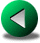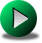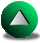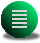Previous: Finite Element Models
Next: Summary
Up: Presentation of the Numerical

Subsections

# The Discontinuous Spectral Element Method

## Introduction

The first development of the spectral element (SE) method occurred in the early eighties [Patera, 1984]. The SE method allows for irregular geometries and high accuracy because of varying order polynomials inside quadrangles or triangles that form the mesh. The main distinction between the FE and SE methods stems from the type of basis functions used to approximate the model equations. In FE methods, the basis functions are usually constructed for one specific order of the scheme (they are derived from Lagrange interpolators on regular grids inside each element). They need to be recomputed as the order of the FE method is modified. In SE methods, the basis functions are hierarchical and follow easier rules of construction (for instance, they can be derived from Chebyshev or Legendre polynomials). As the order is augmented, the former set of basis functions is simply augmented by a new set of polynomials constructed from the previous set. Therefore, in SE methods, the order of approximation is user-dependent and can even vary from element to element. There seem to be numerical advantages in terms of matrix inversion in using the Chebyshev or Legendre polynomials instead of regularly spaced Lagrange interpolators. The latter lead to poorer conditioned matrices as the order of the scheme is augmented [Le Provost and Vincent, 1986]. As with the spectral method, the accuracy of SE method is exponential with increasing polynomial order. However, the SE method offers much higher flexibility in terms of geometrical representation. And, contrary to the spectral method for which Gibbs oscillations are prone to occur in under-resolved regions, in the SE method, one can easily increase the polynomial order (p-refinement) or the number of elements (h-refinement) in the underesolved regions. Using a polynomial order greater than two, we can also expect that SE methods are more accurate than conventional FD or FE methods, and that the convergence of the solution with increasing resolution is much faster.

We noted two applications in ocean modelling using quadrangular SE. The first by Ma ma93 and the second and more successful by Iskandarani and Haidvogel iskan95. Using quadrangles, it is relatively easy to construct an orthogonal basis of cardinal functions which greatly facilitates the computation of nonlinear terms and renders trivial the matrix problem to be solved, provided the equations are prognostic and solved explicitly in time (leapfrog, Adams-Bashforth, Runge-Kutta). One limitation, however, of using continuous basis functions for the primitive (or shallow water) variables is that for stability the maximum polynomial order for approximating pressure (or elevation) has to be lowered, compared to velocity [Iskandarani and Haidvogel, 1995]. Lowering the maximum polynomial order of one variable is similar to staggering the variables in space in finite difference methods and is also similar to satisfying the LBB condition for finite element methods (see previous section). On the other hand, the method leads to a large but sparse matrix problem if the equations are solved implicitly in time, or if a Helmholtz or Poisson-type of system has to be solved. The only disadvantage of using quadrangles compared to triangles comes from the difficulty of discretizing an irregular domain into quadrangles, the triangles offering more flexibility. Using triangles [Sherwin and Karniadakis, 1996], there is no orthogonal basis of cardinal functions. Therefore, a large matrix problem has to be solved at each time-step, even when the equations are discretized explicitly in time. Moreover, the computation of nonlinear terms requires a tedious transfer from the spectral coefficients to values at Gauss-like points, and back to the spectral space. However, in restricted applications, recent developments led to simpler and cheaper algorithms. Lomtev and Karniadakis lomtev99 (hereafter referred as LK) avoid the difficult problem of defining a set of continuous high order polynomials over triangles by reverting to a discontinuous formulation which leads to a local matrix problem in each element-triangle. This is only possible if all the equations are prognostic (as they are for shallow water models) and treated explicitly in time. Luckily, a hydrostatic Boussinesq ocean with a free-surface can be modeled using this simplified spectral element method. Furthermore, their model appears to be stable although the same set of basis functions is used for the velocity and pressure. Thus, their method does not comply to the LBB condition. Finally, this method allows for an easy implementation of a time-variable mesh that we introduce in Section 2.4.3.

## The Model Formulation

The matrix problem to be solved in each element is rather small for the order of the spectral element we choose to test (between 3 to 7). Therefore, the constraint of orthogonality over the set of polynomials for a cost-effective model is made less stringent. Thus, we introduced an even simpler set of basis functions compared to LK by simply using a set of products of Legendre polynomials with a triangular truncation.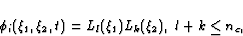(2.47)

where nc is the maximum order of the polynomials and i is indexed as l runs from 0 to nc and k runs from 0 to nc-l. The solution can be expressed inside the element j by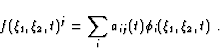(2.48)

For the elements sharing a side with the boundary, the projection of the basis functions onto another set of basis functions which are always zero right at the boundary ensures the different possible boundary conditions (no-normal flow, free-slip, no-slip or inviscid)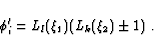(2.49)

The projection method consists of computing coefficients in the new basis using the relation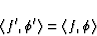(2.50)

which satisfies a least square fit and where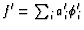. Since the equations are expressed in terms of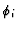, the ai'coefficients of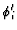have to be expressed in terms ai of. This is straightforward using (2.49). The different boundary conditions can also be implemented for elements sharing only one vertex with the wall. In a square domain, the convergence of the accuracy with resolution was seemingly good with the condition implemented for only elements sharing one side with the wall. Therefore, we only impose the boundary conditions on elements sharing a face with the boundary although some tests were done to investigate this point. Furthermore, in opposition to the continuous spectral element formulations, we stress that the same polynomial order is used for all the variables. From our experience, we never encounter a problem related to the stability, except for trivial CFL problems.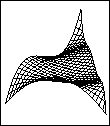We apply the discontinuous spectral element method to the discretization of the shallow water equations. Using a weak formulation and the traditional notation of Galerkin methods, inside each element-triangle the system of equations reads: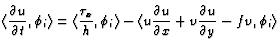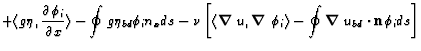(2.51)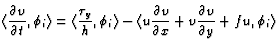(2.52)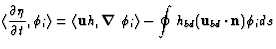(2.53)

where variables and parameters are given in Table 2.1. The line-integrals are very important because they alone transfer information in and out of each element. LK chose to solve a local Riemann problem to compute the boundary value but this technique, being similar to an upwind method, leads to a loss of accuracy. We favored the simple choice of the mean value of both sides of a face which does not affect accuracy. The nonlinear terms are rather expensive to compute (30% of the cost at nc=5). They require a transformation of the local spectral coefficients to a local set of Gaussian points used afterwards to transfer back to the spectral space. The choice of the right Gaussian points is obviously important. After a few trials, we favor the use of irregular points on the triangle [Lyness and Jespersen, 1975,Dunavant, 1985], which are unfortunately only given for polynomials of degree up to 20 (the mass matrix can be exactly computed for). For higher degrees, it is always possible to use a regular set of Legendre-Gauss or Legendre-Lobatto points (but at a higher cost since these sets of points are not optimal on the triangle). For nc=0, we note that the discontinuous SE formulation is equivalent to a FV method.

The time integration is done using a 4th order Runge-Kutta method. Thus, using polynomials of degree nc=5 for instance, gives a certain equivalence between spatial and time truncation errors. The spectral element model is hereafter referred to as SPOC.

A constant eddy viscosity coefficient is used to allow for easy comparisons between models. In a discontinuous spectral element model, the Laplacian operator of the velocity components cannot be computed directly (see LK for details). The computation has to be done in two steps. First, the gradient tensor of the velocity is computed using a weak formulation and an integration by parts. The mass matrix is then inverted: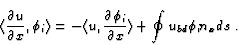(2.54)

Second, the gradient of gradient terms is computed in the momentum equations again using an integration by parts. This ensures that the gradient terms are (weakly) continuous between elements. Since the computation of the gradient tensor is necessary for the computation of the nonlinear terms, this treatment of the diffusion terms does not hamper the computational cost. In 2D, it involves the computation of 4 extra-terms, and in 3D, 9 terms. For the free-slip boundary condition (the one used hereafter), the normal velocity component and the normal derivative of the tangential velocity must vanish at the wall (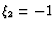). This requires a rotation of the velocity components and of the gradient tensor and a projection onto the special basis function defined in (2.49).

Given the two to three orders of magnitude difference between the scale of eddies and the basin scale, today's global ocean eddy resolving models require a variable in time and space resolution. To fulfill this constraint, not only do we need a variable in space resolution model (which the FE and SE models already offer), but we also need some flexibility of the mesh in time, since eddies and fronts are unsteady phenomena. By adaptive mesh refinement, we mean that the mesh is refined automatically as the simulation goes on in regions where estimated errors are the largest. The difficulty is in computing an error estimator that determines where to put more resolution. For FE methods using linear basis functions, it is usually recommended to estimate the local second order derivatives of the fields and put more resolution where these derivatives are the largest (Zienkiewicz and Taylor, 1991, p.571). Because the solution is piecewise linear, it is difficult to estimate its second order derivatives. This usually requires the reconstruction of the solution by a higher order method [Zhu and Zienkiewicz, 1990]. For continuous SE methods, adaptive strategies require to estimate the slope of the spectral coefficients with wave number. If there is too much energy in the high wave numbers, the elements have to be refined [Mavriplis, 1994]. This is a less complex procedure than that for FE methods. Adaptive strategies are difficult to implement in FD models because the Cartesian grids lack the flexibility of irregular meshes of FE and SE methods. Some adaptive mesh strategies have been proposed, though, in the form of nested grids. The coarse grid follows the overall circulation while the finer grid focuses on a particular region of interest. Both interacting in a one-way or two-way fashion depending on the models [Blayo and Debreu, 1999,Wadley and Bigg, 1999].

From the point of view of defining an error estimator, the discontinuous SE method is slightly more effective. Since the proposed SE formulation allows the solution to be discontinuous between elements, a straightforward estimator is to compute the maximum jump between elements for each field. Though very simple, this estimator has not yet been found in the literature. This is therefore our own development. Once the error estimator has been defined, the refinement or derefinement of the mesh is fairly conventional and can be found in many textbooks, for instance in Zienkiewicz and Taylor (1991) at p.574. We finally end up with four parameters that control the refinement in time (see Table 2.2). The refinement is hierarchical. When a triangle is to be refined, it is cut into four children-triangles and if the neighboring triangles are not to be refined, they are cut into two children-triangles in order to have a conformal connectivity. But if one of the two children-triangles is to be later refined, their parent-triangle will be cut into four, as the cutting into two children-triangles is only needed to complete the connectivity (Fig.2.12). All the refinements of the mesh will be kept in memory, easing the backward process of derefinement. The CFL condition is updated every time the mesh is modified. The model requires a certain adjustment time in order to smooth the jump between elements after each refinement of the mesh. Therefore there is a minimum value for ncheck (Table 2.2) depending on the time-step and the physical parameters. Hence, for a time stepping simulation, the model stops regularly to check the level of errors, refines the resolution accordingly, interpolates the fields onto the new mesh and then restarts with the new mesh and fields. In contrast to steady flows for which the solution is unique (if the initial guess is close enough), the transient simulations present the disadvantage that the solution accuracy might degrade because the errors are still present in the new fields, although the resolution has been improved. This justifies the use of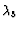, the relative jump value above which the errors have reached an unacceptable level. If so, the model should not restart from the present time-step but from a previously saved time-step at which the level of errors was acceptable. The question of accuracy of adaptive time-stepping solutions also arises from the issue of interpolating the variable fields, since the interpolation does not conserve mass or energy.

Table 2.2: Refinements parameters used in the simulations unless otherwise specified.
 Para-meters Relative value Function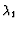0.01-0.03 value of the jump above which the element is refined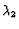0.001 value of the jump below which the element is to be derefined0.05-0.15 value of the jump above which the simulation is restarted using older fields ncheck 1000 number of time step between two checks of the jumps between the elements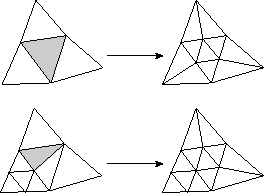## Curved Spectral Element Method

As we stressed in Section 2.2, the representation of the irregular geometry is the weakest point of FD methods. They represent the coastline as step-like walls. This would be equivalent to say that the boundary is piecewise constant, i.e, discontinuous. In contrast, FE methods usually represent a complex boundary by piecewise linear segments. Thus, the model boundary is C0 continuous. In order to represent accurately a complex boundary in SE formulations, it is better to stretch or curve the element boundaries than to increase the number of elements in a region of strong curvature (and keep the model boundary piecewise linear) as done in FE methods. Doing otherwise results in an increase in the number of elements and an increase in the resolution to the point that the cost of using higher order polynomials becomes prohibitive. It makes more sense to take advantage of the high order to get a boundary as smooth as possible (and try to get rid of discontinuities between piecewise segments along the boundary). This allows for faster convergence rates when the numerical solution is compared to analytical solutions found in continuously varying curvature domains. Furthermore, high order methods tend to behave badly in the presence of singularities along the boundaries (Gibbs oscillations). This is particularly true for this discontinuous SE method. In fact, we observe in one test-experiment these oscillations localized around the tip of one rectangular continent. Hence, a clear limitation of this SE formulation lies in the presence of singularities along the coastline. It is not so much a surprise that the high order methods tends to behave badly in the presence of singularities compared with low order methods. Singularities excite the highest modes of the high order methods and so, lead to strong oscillations. In particular, the adaptive method developed in the previous chapter fails to convergence in the presence of singularities (not shown). Therefore, the solution may come from smoothing out the geometry by using curved elements. In practice, the additional cost associated with the implementation of curved elements in triangular spectral elements limits the order of continuity of the model boundary. This section is devoted to the development of a curved spectral element model. Although curved spectral elements may appear natural, few details are available in the literature about their implementation. We therefore develop our own methodology.For a triangle with local coordinates varying in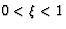,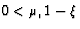, there is a local analytical transformation that transforms one of the faces into a parabolic segment (Fig. 2.13):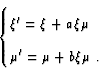(2.55)

The segment is parabolic in the sense that it can be represented by an equation which is quadratic in term of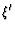and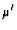. Hence, we can represent curved coastlines as piecewise parabolic segments. Since the coordinate system we choose for integration over the triangle (Fig. 2.14) is,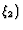, the exact transformation is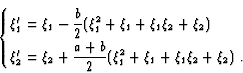(2.56)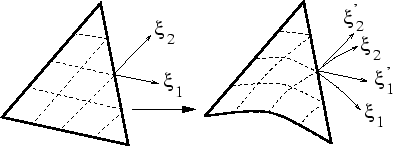The Jacobian matrix J of this transformation is needed for computing the integrals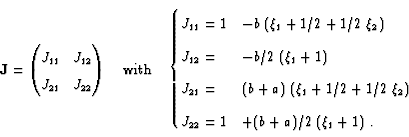(2.57)

For instance the computation of the mass matrix M becomes(2.58)

The obvious inconvenience is that the Gaussian rules we use to compute the integrals and, more specifically, the nonlinear terms, need to be augmented by two degrees, since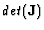is a polynomial expression of degree 2. Therefore, a set of polynomials of degree 5 require a Gaussian rule of degree 12 instead of 10. Keeping the old set of Gaussian rule is not impossible but leads to large errors since the integrals are not exactly evaluated. From that point of view, the spectral quadrangle is more efficient. Since it exists a set of cardinal-orthogonal polynomials on a rectangle, it is more effective to keep the old set of Gauss-Lobatto points even if the integrals are no more exact in a curved quadrangle. In fact, the errors in the computation of the integrals are, in this case, roughly of the order of the maximum polynomial order [Ronquist, 1980]. However, for triangular spectral elements, the inconvenience of increasing the number of Gaussian points applies only for the curved elements along the boundary. Therefore, the problem of additional cost is not so serious since it concerns a small set of elements.Previous: Finite Element Models
Next: Summary
Up: Presentation of the Numerical

Frederic Dupont
2001-09-11